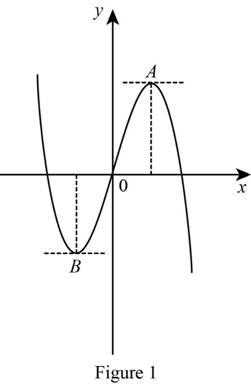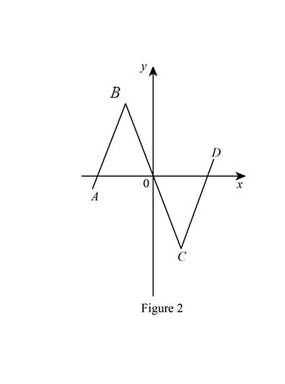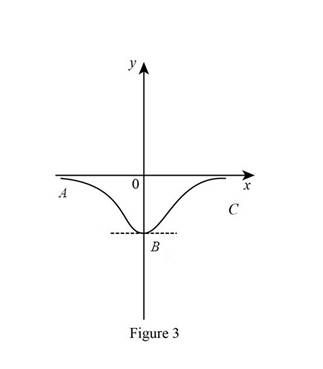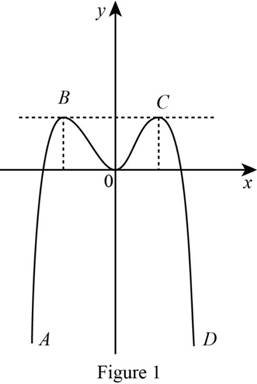# To match: The graph of f with graph of its derivative with proper explanation.### Single Variable Calculus: Concepts...

4th Edition
James Stewart
Publisher: Cengage Learning
ISBN: 9781337687805### Single Variable Calculus: Concepts...

4th Edition
James Stewart
Publisher: Cengage Learning
ISBN: 9781337687805

#### Solutions

Chapter 2.7, Problem 3E

(a)

To determine

## To match: The graph of f with graph of its derivative with proper explanation.

Expert Solution

The derivative of the graph (a) is graph II.

### Explanation of Solution

Given:

The given graph of the function f is shown in Figure 1.Observation:

From the Figure 1, it is observed that the graph of f contains the horizontal tangents at two points. Let the two points be A and B.

Note that, the value of the derivative will be zero at the point where the function has the horizontal tangent.

So, the graph of f will be zero at the points A and B.

Thus, the derivative of the graph will have two zeros at x coordinate where

curve f has horizontal tangent. (1)

The curve in section AB has positive slope which implies that the curve of derivative

at that section will have positive value. (2)

The condition mentioned in (1) and (2) is satisfied by graph II.

Thus, the derivative of the graph (a) is graph II.

(b)

To determine

### To Match: The graph of of f with graph of its derivative with proper explanation.

Expert Solution

The derivative of the graph (b) is the graph IV.

### Explanation of Solution

Graph:

The given graph of the function f is shown in Figure 2.Observation:

From the graph, it is observed that the graph has a corner point at two points.

Let the two points be A and B. The derivative graph f is discontinuous at these points. Thus, the graph of derivative at points A and B does not exist. (3)

The graph from point A to B has negative slope which implies that the derivative graph must have negative value in this section. Since the function is linear in this part, the derivative graph will be horizontal line.

Thus, the derivative graph will be horizontal line below y axis. (4)

The condition mentioned in (3) and (4) is satisfied by graph IV.

Thus, the derivative of the graph (b) is graph IV.

(c)

To determine

### To match: The graph of f with graph of its derivative with proper explanation.

Expert Solution

The derivative of the graph (c) is the graph I.

### Explanation of Solution

Graph:

The given graph of the function f is shown in Figure 3.Observation:

From the Figure 3, it is observed that the graph of f contains the horizontal tangent at one point. Let the point be B.

Note that, the value of the derivative will be zero at the point where the function has the horizontal tangent.

So, the graph of f will be zero at the point A. (5)

The curve in section AB has negative slope which implies that the curve of derivative

at that section will have negative value. (6)

The curve in section BC has positive slope which implies that the curve of derivative

at that section will have positive value. (7)

The condition mentioned in (5), (6) and (7) is satisfied by graph I.

Thus, the derivative of the graph (c) is graph I.

(d)

To determine

### To match: The graph of f with graph of its derivative with proper explanation.

Expert Solution

The derivative of the graph (d) is the graph III.

### Explanation of Solution

Graph:Observation:

From the Figure 4, it is observed that the graph of f contains the horizontal tangents at two points. Let the two points be B and C.

Note that, the value of the derivative will be zero at the point where the function has the horizontal tangent.

So, the graph of f will be zero at the points B and C.

Thus, the derivative of the graph will have two zeros at x coordinate where

curve f has horizontal tangent.

From the Figure 2, it is observed that the curve left to right, the slope of the tangents to the graph are positive then becomes zero, then negative, then zero followed by zero and becomes positive.

The graph III has the same pattern as described above.

Thus, the derivative of the graph (d) is graph III.

### Have a homework question?

Subscribe to bartleby learn! Ask subject matter experts 30 homework questions each month. Plus, you’ll have access to millions of step-by-step textbook answers!